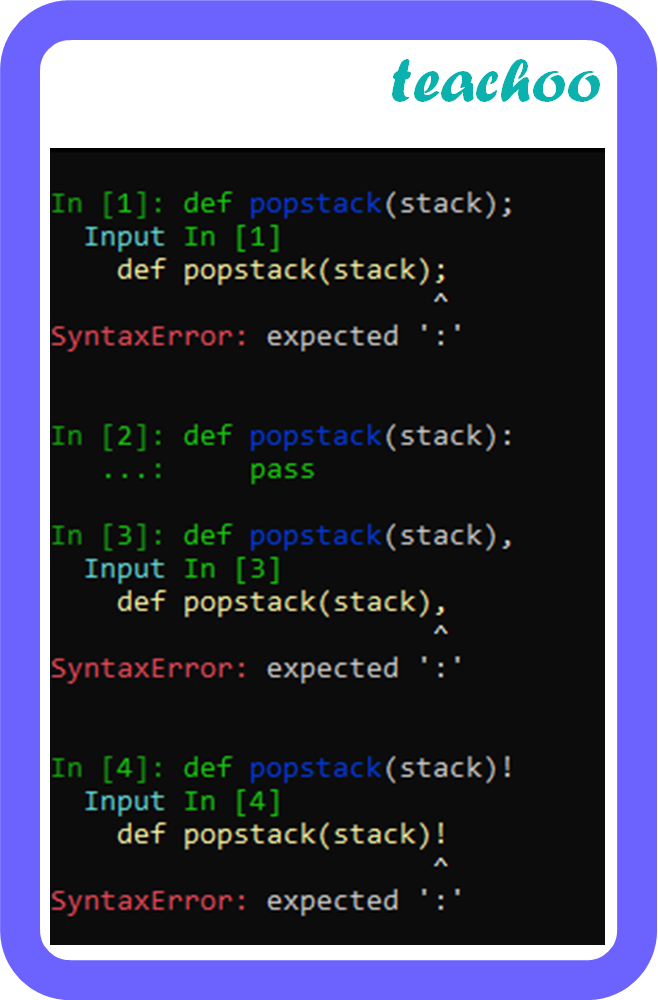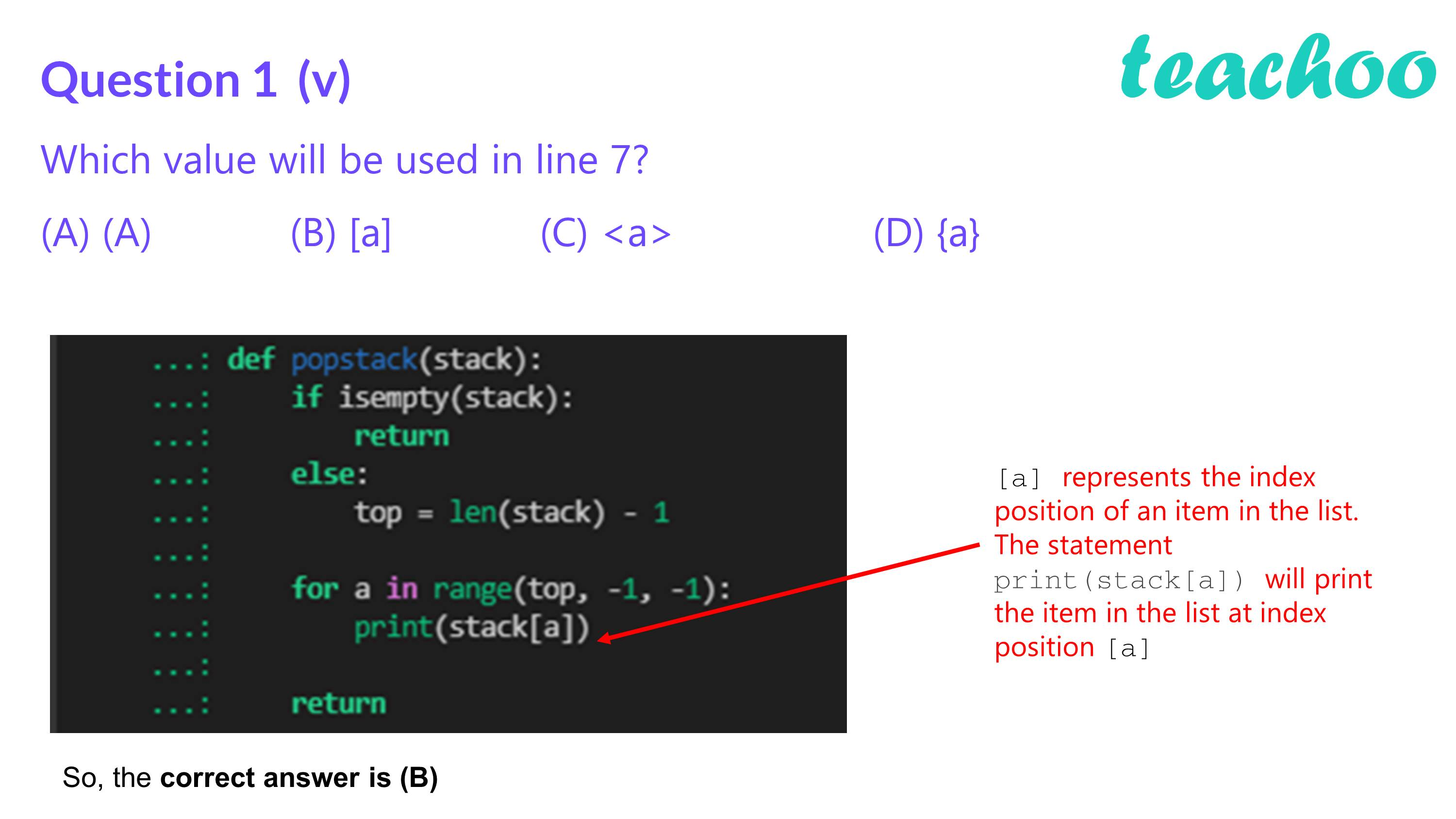Case Based MCQ Questions

Computer Science - Class 12
Chapter 3 - Stacks

## (A) ;   (B) :    (C) ,  (D) !

In python, a colon (:) is used to mark the beginning of a function.Checking all the options, we note that all give errors except one

So, the correct answer is (B)

##Learn in your speed, with individual attention - Teachoo Maths 1-on-1 Class

### Transcript

Here is the code for pop method in stack to print a string in reverse order. But there is some missing word. Answer the questions that follow to execute the code successfully. def popstack (stack)__ #Line 1 if isempty (stack): #Line 2 ______ #Line 3 ______ : #Line 4 top = len(stack) __ #Line 5 for a in range (top, –1, –1): print stack__, #Line 7 return Question 1 (i) Which symbol is used to terminate the function popstack()? (A) ; (B) : (C) , (D) ! Answer: In python, a colon (:) is used to mark the beginning of a function. Checking all the options, we note that all give errors except one So, the correct answer is (B) Question 1(ii) Fill the blank in line 3 (A) return (B) True (C) False (D) 0 Answer: Question 1(iii) Which variable or keyword will be best suited in fill the blank in line 4? (A) elif (B) for (C) while (D) else Answer: Question 1(iv) Fill the blank in line 5 (A) 0 (B) 1 (C) 2 (D) –1 Answer: Question 1(v) Which value will be used in line 7? (A) (A) (B) [a] (C) <a> (D) {a} Answer: